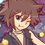# Combinatorics (1st math Thailand POSN 2014)

1.) Answer with factorial notations and simplify into numbers.

• 1.1) Find the coefficients of $x^{5}$ from the expansion of $(2+x+x^{2})^{8}$
• 1.2) There're 10 people, including A,B,C,D. If we set them arrange in the long bench, find the number of different ways are there, such that only 2 of A,B,C,D are sitting together.

2.) Prove these statements by combinatorial proof.

• 2.1) $\displaystyle \sum\limits_{k=0}^{r} \dbinom{r}{k}^{2} = \dbinom{2r}{r}$ for any natural number $r$.

• 2.2) $\displaystyle \frac{(9n)!}{2^{5n}3^{n}}$ is always integer for any natural number $n$.

3.) Throw 15 6-sided regular dice, find the number of different ways such that every 6 different sides are shown and no more than 3 same sides are shown.

4.) Let $V(r,n)$ be the number of ways of putting $r$ different objects into $n$ identical boxes such that each boxes must have at least $k$ objects Prove that

$V(r,n) = nV(r-1,n) + \dbinom{r-1}{r-k}V(r-k,n-1)$

for any natural number $r,n,k$ and $r \geq nk$.

5.) There was a rumour inside the group of 10 people. This rumour is spread by e-mail and continuously spread by following rules.

• First, there was only 1 people know about the rumour called rumour-er.
• Each e-mail can be either forwarded directly (exactly 1 people and can forward again) or people who receive copies (can be 0 people or any number of people, but cannot forward again)
• People who received the e-mail (by both ways) can know who sent the mail, and those are considered to be rumoured
• People who received by forwarding can only forward the mail once and only forward to people who are not rumoured.
• Rumour-er can send as many e-mails as he/she want.

How many ways are there if the e-mails are sent exactly 2 times, and how many ways if sent exactly 3 times?

This is the part of Thailand 1st round math POSN problems.Note by Samuraiwarm Tsunayoshi
6 years, 10 months ago

This discussion board is a place to discuss our Daily Challenges and the math and science related to those challenges. Explanations are more than just a solution — they should explain the steps and thinking strategies that you used to obtain the solution. Comments should further the discussion of math and science.

When posting on Brilliant:

• Use the emojis to react to an explanation, whether you're congratulating a job well done , or just really confused .
• Ask specific questions about the challenge or the steps in somebody's explanation. Well-posed questions can add a lot to the discussion, but posting "I don't understand!" doesn't help anyone.
• Try to contribute something new to the discussion, whether it is an extension, generalization or other idea related to the challenge.

MarkdownAppears as
*italics* or _italics_ italics
**bold** or __bold__ bold
- bulleted- list
• bulleted
• list
1. numbered2. list
1. numbered
2. list
Note: you must add a full line of space before and after lists for them to show up correctly
paragraph 1paragraph 2

paragraph 1

paragraph 2

[example link](https://brilliant.org)example link
> This is a quote
This is a quote
    # I indented these lines
# 4 spaces, and now they show
# up as a code block.

print "hello world"
# I indented these lines
# 4 spaces, and now they show
# up as a code block.

print "hello world"
MathAppears as
Remember to wrap math in $$ ... $$ or $ ... $ to ensure proper formatting.
2 \times 3 $2 \times 3$
2^{34} $2^{34}$
a_{i-1} $a_{i-1}$
\frac{2}{3} $\frac{2}{3}$
\sqrt{2} $\sqrt{2}$
\sum_{i=1}^3 $\sum_{i=1}^3$
\sin \theta $\sin \theta$
\boxed{123} $\boxed{123}$

Sort by:

3) You are looking for the number of solutions to

$a + b + c + d + e + f = 15$, subject to $1 \leq a \leq 3$, and similarly for all the other variables.

Hint: Use the substitution $z = 3 - a$.

Staff - 6 years, 10 months ago

Wow, this is nice! Thank you ^_^

- 6 years, 10 months ago

2.) I use multiset to prove, but I forgot how to form a multiset.

- 6 years, 10 months ago

$M =\{ 4n\cdot a_{1}, 2n\cdot a_{2}, 2n\cdot a_{3}, 1n\cdot a_{4}\}$ such that $|M| = 9n$.

Number of permutations = $\displaystyle \frac{(9n)!}{(4!)^{n}(2!)^{n}(2!)^{n}(1!)^{n}} = \frac{(9n)!}{2^{5n}3^{n}}$ which is always integer. Done!

I swear to goat that I haven't done this for years since the last year test.

- 6 years, 10 months ago